Next: Rectangular Coordinates. Finite Bodies, Up: Rectangular Coordinates. Steady 1-D. Previous: Semi infinite body, steady

X11 Plate, G = 0 (Dirichlet) at x = 0 and x = L.

 GX11(x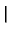x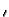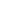) =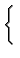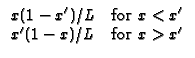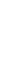X12 Plate, G = 0 (Dirichlet) at x = 0 and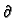G/x = 0 (Neumann) at x = L.

 GX12(xx) =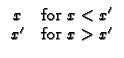X13 Plate, G = 0 (Dirichlet) at x = 0 and kG/x + h2G = 0 (Neumann) at x = L Note B2 = h2L/k

 GX13(xx) =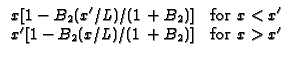X21 Plate,G/x = 0 (Neumann) at x = 0 and G = 0 (Dirichlet) at x = L.

 GX21(xx) =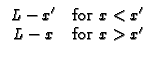X22 Plate,G/x = 0 (Neumann) at both sides. Note that this geometry requires a pseudo GF, denoted H. The temperature solution found from a pseudo GF requires that the total volumetric heating sums to zero and the spatial average temperature in the body must be supplied as a known condition.

 HX22(xx) =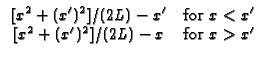X23 Plate,G/x = 0 (Neumann) at x = 0 and kG/x + h2G = 0 (convection) at x = L. Note B2 = h2L/k

 GX23(xx) =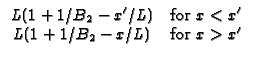X31 Plate, - kG/x + h1G = 0 (convection) at x = 0 and G = 0 (Dirchlet) at x = L. Note B1 = h1L/k

 GX31(xx) =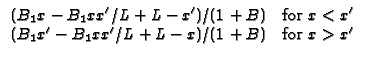X32 Plate, - kG/x + h1G = 0 (convection) at x = 0 andG/x = 0 (Neumann) at x = L. Note B1 = h1L/k

 GX32(xx) =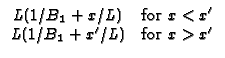X33 Plate, - kG/x + h1G = 0 (convection) at x = 0 and kG/x + h2G = 0 (convection) at x = L. Note B1 = h1L/k and B2 = h2L/k
 GX33(xx) =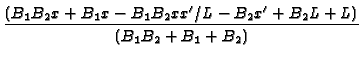; forx < x=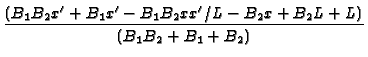; forx > xNext: Rectangular Coordinates. Finite Bodies, Up: Rectangular Coordinates. Steady 1-D. Previous: Semi infinite body, steady
Kevin D. Cole
2002-12-31